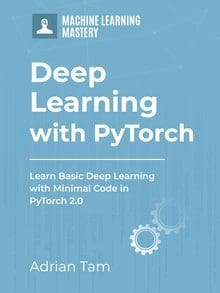# Multi-Target Predictions with Multilinear Regression in PyTorch

While in the previous few tutorials we worked with single output multilinear regression, here we’ll explore how we can use multilinear regression for multi-target predictions. Complex neural network architectures are essentially having each neuron unit to perform linear regression independently then pass on their result to another neuron. Therefore, knowing how such regression works is useful to understand how a neural network performs multi-target predictions.

The goal of this article is to provide a step-by-step guide for the implementation of multi-target predictions in PyTorch. We will do so by using the framework of a linear regression model that takes multiple features as input and produces multiple results.

We will start by importing the necessary packages for our model. We will then define our input data points and what we want to achieve with our model. Particularly, we’ll demonstrate:

• How to understand multilinear regression in multiple dimensions.
• How to make multi-target predictions with multilinear regression in PyTorch.
• How to build class linear using the ‘nn.Module’ in PyTorch.
• How to make multi-target predictions with a single input data sample.
• How to male multi-target predictions with multiple input data samples.

Note that we’ll not train our MLR model in this tutorial, we’ll only see how it makes simple predictions. In the subsequent tutorial of our PyTorch series, we’ll learn how this model can be trained on a dataset.

Kick-start your project with my book Deep Learning with PyTorch. It provides self-study tutorials with working code.

Let’s get started.Multi-Target Predictions with Multilinear Regression in PyTorch.
Picture by Dan Gold. Some rights reserved.

## Overview

This tutorial is in three parts; they are

• Create the Module
• Making Predictions with Sinple Input Samples
• Making Predictions with Multiple Input Samples

## Create the Module

We’ll build a custom linear class for our multilinear Regression model. We’ll define a linear class and make it a child class of the PyTorch package nn.Module. This class inherits all the methods and attributes from the package, such as nn.Linear.

Now, let’s create the model object and define the parameters accordingly. As we plan on making multi-target predictions, let’s first check how our model works for a single input sample. Later, we’ll make predictions for multiple input samples.

## Making Predictions with Single Input Samples

We’ll create our model object that takes a single input sample and makes five predictions.

Now, lets define our input tensor x for the model and make predictions.

Here’s what the output looks like.

As you can see, our model made multiple predictions out of only a single input sample. Here is how we can list the model parameters.

and the output is like the following:

You may get a different result in numbers as those are randomized weights, but the shape of the weight tensors would match our design of taking one input and giving five output.

### Want to Get Started With Deep Learning with PyTorch?

Take my free email crash course now (with sample code).

Click to sign-up and also get a free PDF Ebook version of the course.

## Making Predictions with Multiple Input Samples

Similarly, let’s define a tensor X for multiple input samples, where each row represents a data sample.

We can make multi-target predictions with multiple input samples.

As we have three samples of input, we should see three samples of output, like the following:

Putting everything together, the following is the complete code:

## Summary

In this tutorial, you learned how you can make multi-target predictions with multilinear regression model. Particularly, you learned:

• How to understand multilinear regression in multiple dimensions.
• How to make multi-target predictions with multilinear regression in PyTorch.
• How to build class linear using the ‘nn.Module’ in PyTorch.
• How to make multi-target predictions with a single input data sample.
• How to male multi-target predictions with multiple input data samples.

## Get Started on Deep Learning with PyTorch!#### Learn how to build deep learning models

...using the newly released PyTorch 2.0 library

Discover how in my new Ebook:
Deep Learning with PyTorch

It provides self-study tutorials with hundreds of working code to turn you from a novice to expert. It equips you with
tensor operation, training, evaluation, hyperparameter optimization, and much more...